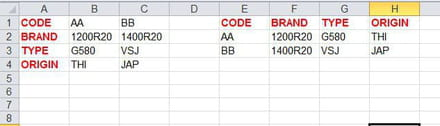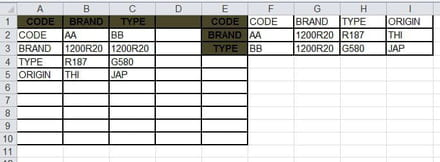# Transpose data from vertical to horizontal

Solved/Closed
abdelfatah_0230
Posts
73
Registration date
Thursday July 18, 2019
Status
Member
Last seen
July 23, 2022
- Jun 11, 2020 at 05:28 AM
abdelfatah_0230
Posts
73
Registration date
Thursday July 18, 2019
Status
Member
Last seen
July 23, 2022
- Jun 16, 2020 at 02:38 PM
Hello,
this the code doesn't work i would transpose as what in existed in picture the result from e: h i hope somebody help me
`Sub VerticalToHorizontal()  Dim a As Variant, b As Variant  Dim RowsPerBlock As Long, NumBlocks As Long, i As Long, j As Long, BaseNum As Long    RowsPerBlock = Columns(1).Find(What:=Range("A1").Value, LookAt:=xlWhole).Row - 1    '*** RowsPerBlock will =1 if A1 is empty  or   '*** it will = 0 if A1 is the only cell in the column with data  If RowsPerBlock = 0 Or Range("A1") = "" Then    Exit Sub  End If  a = Range("B1:C" & Range("A" & Rows.Count).End(xlUp).Row).Value  NumBlocks = UBound(a) / RowsPerBlock  ReDim b(1 To NumBlocks, 1 To RowsPerBlock)  Do Until i = NumBlocks    i = i + 1    BaseNum = (i - 1) * RowsPerBlock    For j = 1 To RowsPerBlock      b(i, j) = a(BaseNum + j, 1)    Next j  Loop  With Range("e1").Resize(, RowsPerBlock)    .Value = Application.Transpose(Range("A1").Resize(RowsPerBlock).Value)    .Offset(1).Resize(NumBlocks).Value = b  End WithEnd Sub`## 2 replies

Posts
2900
Registration date
Sunday September 12, 2010
Status
Moderator
Last seen
September 12, 2022
523
Updated on Jun 15, 2020 at 11:55 AM
Hi Abdel,

Your code doesn't make much sense to me. RowsPerBlock will always be 0, since the value of A1 will always be found on the first row of the first column. And when it is 0, the code ends ....

So I took the liberty to write my own code. I hope you don't mind ;).

Here is the code:
```Sub RunMe()
Dim lRow, lCol, x As Integer

lRow = Range("A1").End(xlDown).Row
lCol = Range("A1").End(xlToRight).Column

For Each cell In Range(Cells(1, 1), Cells(1, lCol))
x = x + 1
Range(Cells(1, cell.Column), Cells(lRow, cell.Column)).Copy
Cells(x, lCol + 2).PasteSpecial Transpose:=True
Next cell
Application.CutCopyMode = False
End Sub```

Best regards,
Trowa

abdelfatah_0230
Posts
73
Registration date
Thursday July 18, 2019
Status
Member
Last seen
July 23, 2022

Jun 15, 2020 at 03:36 PM
hi, Trowa
first of all this code is not original it was existed error somebody try fix the error by this RowsPerBlock always be 0
it doesn't solve my problem the secondly i don't mind if is solve my problem your code is nice but i think you should fix as my image in column e it supposes doesn't show and i would dynamically transpose data maybe i have many brands i'm talking about from col a,b maybe data also in col c,d,e..etcPosts
2900
Registration date
Sunday September 12, 2010
Status
Moderator
Last seen
September 12, 2022
523
Jun 16, 2020 at 11:40 AM
Hi Abdel,

Ok, I added a line to clear the transposed data. When you want to add another column of data, right-click the empty column between original and transposed data (so column D for the first extra data column) and insert a column (to keep an empty column between original and transposed data), enter your data and run the code again to update (actually create new) the transposed data.

Here is the updated code:
```Sub RunMe()
Dim lRow, lCol, x As Integer

lRow = Range("A1").End(xlDown).Row
lCol = Range("A1").End(xlToRight).Column

Cells(1, lCol + 2).CurrentRegion.ClearContents

For Each cell In Range(Cells(1, 1), Cells(1, lCol))
x = x + 1
Range(Cells(1, cell.Column), Cells(lRow, cell.Column)).Copy
Cells(x, lCol + 2).PasteSpecial Transpose:=True
Next cell
Application.CutCopyMode = False
End Sub```

PS: When you remove a column of data, you will want to run the code twice to get correct transposed data.

Best regards,
Trowa
abdelfatah_0230
Posts
73
Registration date
Thursday July 18, 2019
Status
Member
Last seen
July 23, 2022

Jun 16, 2020 at 02:38 PM
i noted if there is no empty col between orginal data and transpose data it transposes data to a new cols and copy data incorrectly and if i insert empty col between them it works very well even if i adjust original data .
thanks Trowa for this code it works very well